# Kinetic Energy Formula

Kinetic Energy Formula

Kinetic energy is the energy of moving objects. An object's kinetic energy depends on the object's mass and velocity. The unit of kinetic energy is Joules (J). In terms of other units, one Joule is equal to one kilogram meter squared per second squared (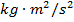).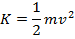K = kinetic energy (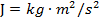)

m = mass (kg)

v = velocity (m/s)

Kinetic Energy Formula Questions:

1) What is the kinetic energy of a 0.500 kg rabbit, running at 8.00 m/s?

Answer: The kinetic energy of the rabbit can be found using the formula: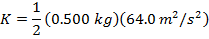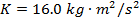K = 16.0 J

The kinetic energy of the rabbit is 16.0 J (Joules).

2) What is the kinetic energy of a truck with mass 3000 kg, traveling at 90.00 km/h?

Answer: The first step to solving the question is to convert the velocity of the truck to meters per second (m/s). It is useful to write the conversion in the following way:

v = 90.00 km/h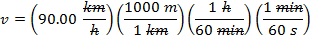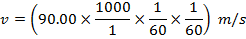v = 25.00 m/s

The kinetic energy of the truck can be found using the formula: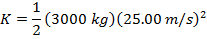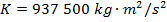K = 937 500 J

The kinetic energy of the truck is 937 500 J (Joules).